# Selina Solutions Concise Maths Class 10 Chapter 13 Section and Mid-Point Formula Exercise 13(B)

The important topics covered under this exercise are mid-point formula and centroid of a triangle. Solving problems of this exercise, students are able to attain a good grip over the concepts mentioned above. The Selina Solutions for Class 10 Maths is a great tool for learning the right steps in solving problems. The solutions of this exercise are available in the Concise Selina Solutions for Class 10 Maths Chapter 13 Section and Mid-Point Formula Exercise 13(B) PDF, which is provided in the links below.

## Selina Solutions Concise Maths Class 10 Chapter 13 Section and Mid-Point Formula Exercise 13(B) Download PDF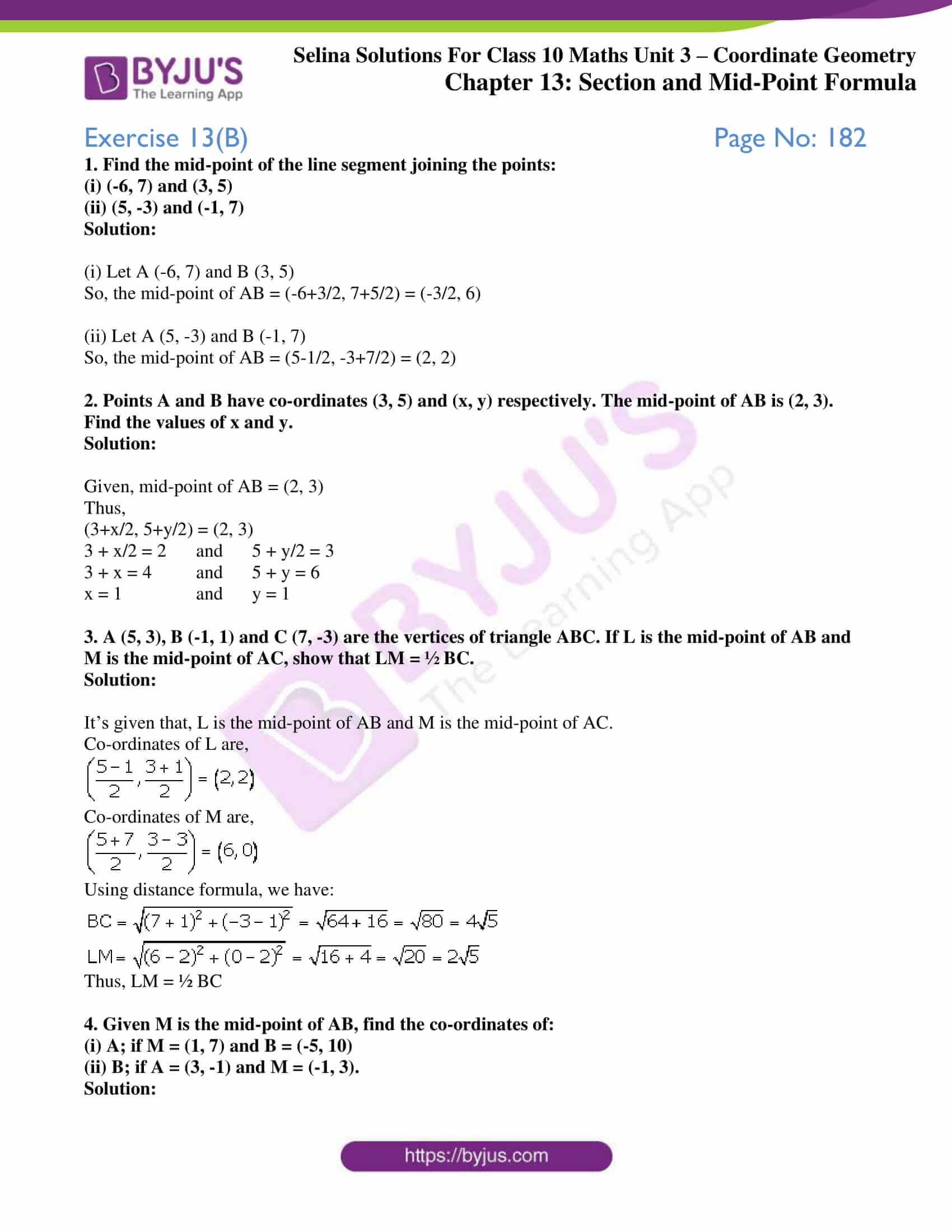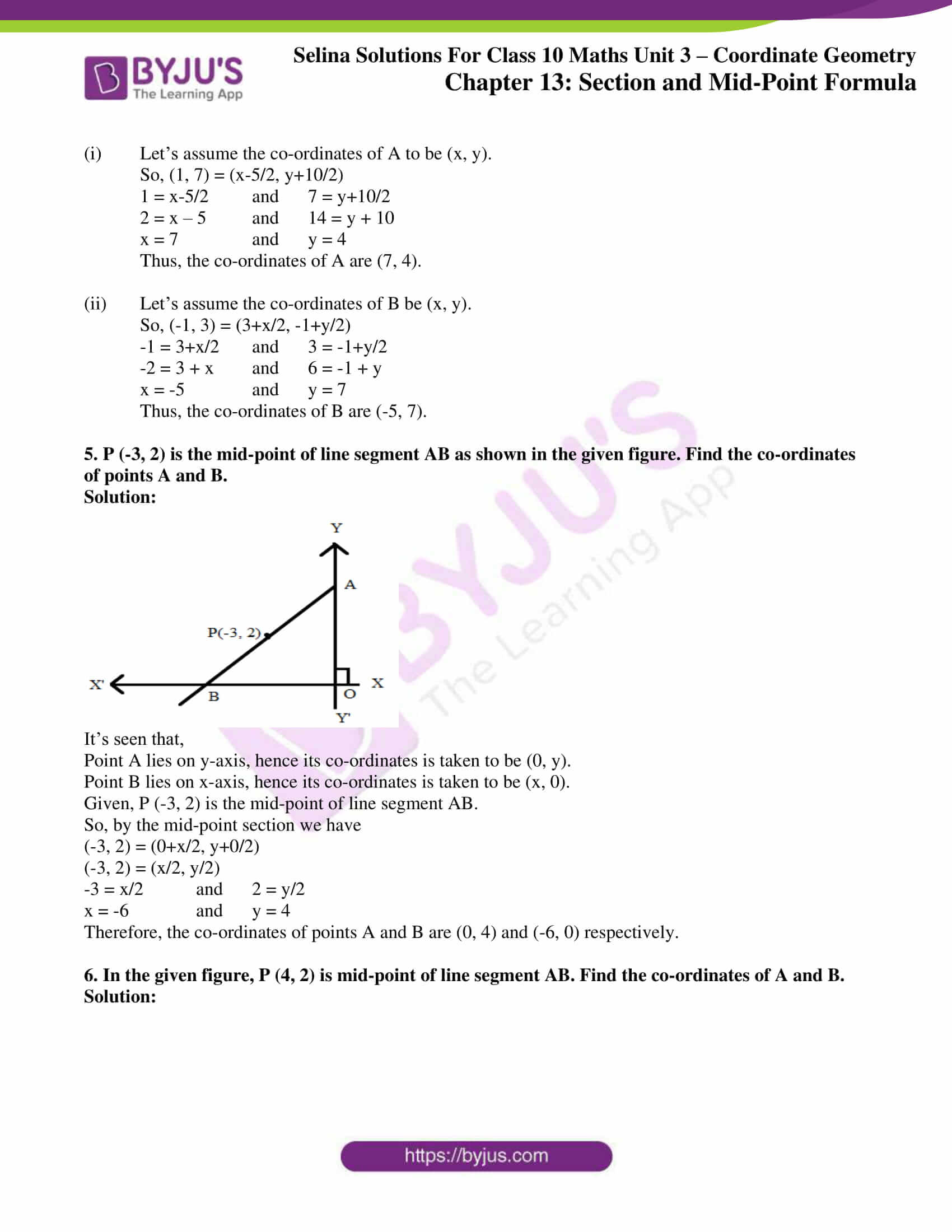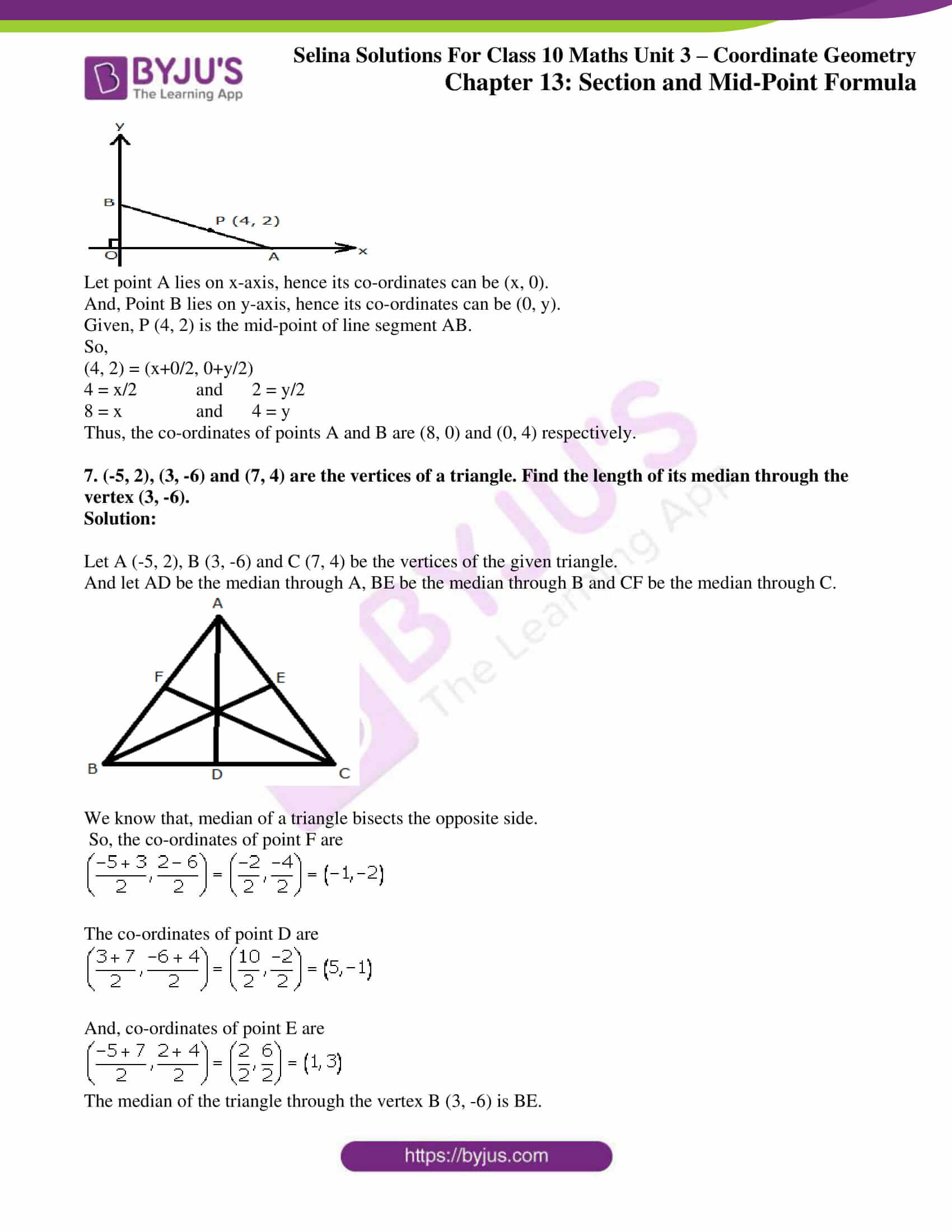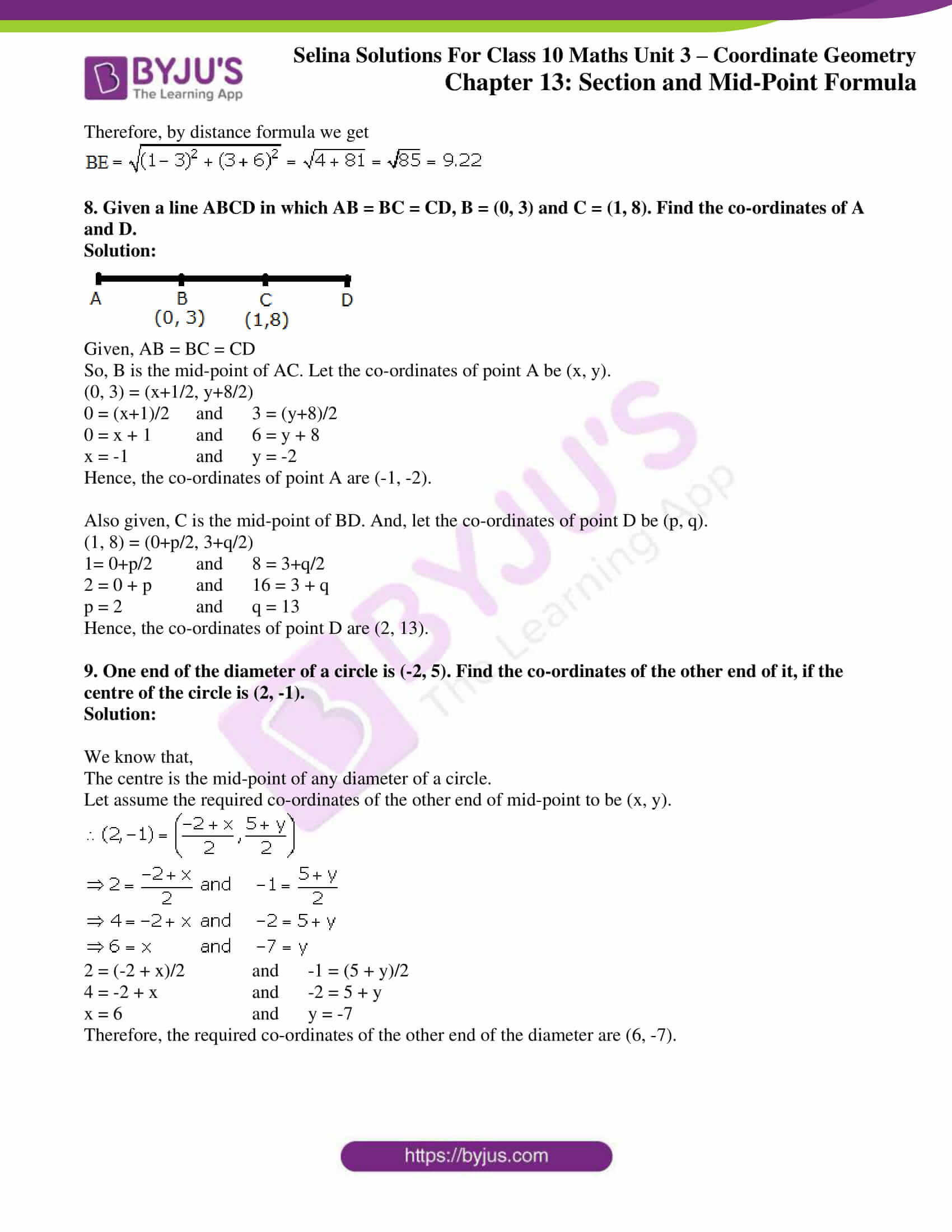### Access Selina Solutions Concise Maths Class 10 Chapter 13 Section and Mid-Point Formula Exercise 13(B)

1. Find the mid-point of the line segment joining the points:

(i) (-6, 7) and (3, 5)

(ii) (5, -3) and (-1, 7)

Solution:

(i) Let A (-6, 7) and B (3, 5)

So, the mid-point of AB = (-6+3/2, 7+5/2) = (-3/2, 6)

(ii) Let A (5, -3) and B (-1, 7)

So, the mid-point of AB = (5-1/2, -3+7/2) = (2, 2)

2. Points A and B have co-ordinates (3, 5) and (x, y) respectively. The mid-point of AB is (2, 3). Find the values of x and y.

Solution:

Given, mid-point of AB = (2, 3)

Thus,

(3+x/2, 5+y/2) = (2, 3)

3 + x/2 = 2 and 5 + y/2 = 3

3 + x = 4 and 5 + y = 6

x = 1 and y = 1

3. A (5, 3), B (-1, 1) and C (7, -3) are the vertices of triangle ABC. If L is the mid-point of AB and M is the mid-point of AC, show that LM = ½ BC.

Solution:

It’s given that, L is the mid-point of AB and M is the mid-point of AC.

Co-ordinates of L are,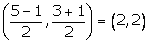Co-ordinates of M are,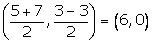Using distance formula, we have: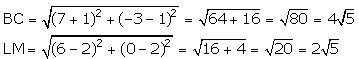Thus, LM = ½ BC

4. Given M is the mid-point of AB, find the co-ordinates of:

(i) A; if M = (1, 7) and B = (-5, 10)

(ii) B; if A = (3, -1) and M = (-1, 3).

Solution:

(i) Let’s assume the co-ordinates of A to be (x, y).

So, (1, 7) = (x-5/2, y+10/2)

1 = x-5/2 and 7 = y+10/2

2 = x – 5 and 14 = y + 10

x = 7 and y = 4

Thus, the co-ordinates of A are (7, 4).

(ii) Let’s assume the co-ordinates of B be (x, y).

So, (-1, 3) = (3+x/2, -1+y/2)

-1 = 3+x/2 and 3 = -1+y/2

-2 = 3 + x and 6 = -1 + y

x = -5 and y = 7

Thus, the co-ordinates of B are (-5, 7).

5. P (-3, 2) is the mid-point of line segment AB as shown in the given figure. Find the co-ordinates of points A and B.

Solution: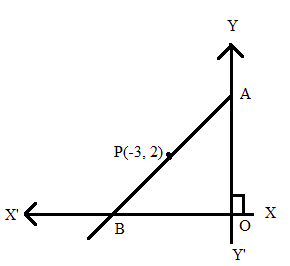It’s seen that,

Point A lies on y-axis, hence its co-ordinates is taken to be (0, y).

Point B lies on x-axis, hence its co-ordinates is taken to be (x, 0).

Given, P (-3, 2) is the mid-point of line segment AB.

So, by the mid-point section we have

(-3, 2) = (0+x/2, y+0/2)

(-3, 2) = (x/2, y/2)

-3 = x/2 and 2 = y/2

x = -6 and y = 4

Therefore, the co-ordinates of points A and B are (0, 4) and (-6, 0) respectively.

6. In the given figure, P (4, 2) is mid-point of line segment AB. Find the co-ordinates of A and B.

Solution: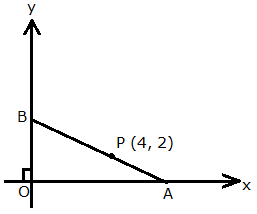Let point A lies on x-axis, hence its co-ordinates can be (x, 0).

And, Point B lies on y-axis, hence its co-ordinates can be (0, y).

Given, P (4, 2) is the mid-point of line segment AB.

So,

(4, 2) = (x+0/2, 0+y/2)

4 = x/2 and 2 = y/2

8 = x and 4 = y

Thus, the co-ordinates of points A and B are (8, 0) and (0, 4) respectively.

7. (-5, 2), (3, -6) and (7, 4) are the vertices of a triangle. Find the length of its median through the vertex (3, -6).
Solution:

Let A (-5, 2), B (3, -6) and C (7, 4) be the vertices of the given triangle.

And let AD be the median through A, BE be the median through B and CF be the median through C.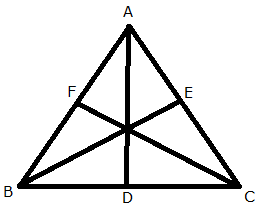We know that, median of a triangle bisects the opposite side.

So, the co-ordinates of point F are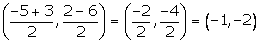The co-ordinates of point D are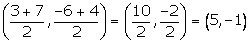And, co-ordinates of point E are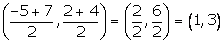The median of the triangle through the vertex B (3, -6) is BE.

Therefore, by distance formula we get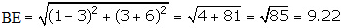8. Given a line ABCD in which AB = BC = CD, B = (0, 3) and C = (1, 8). Find the co-ordinates of A and D.

Solution: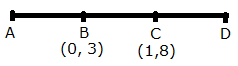Given, AB = BC = CD

So, B is the mid-point of AC. Let the co-ordinates of point A be (x, y).

(0, 3) = (x+1/2, y+8/2)

0 = (x+1)/2 and 3 = (y+8)/2

0 = x + 1 and 6 = y + 8

x = -1 and y = -2

Hence, the co-ordinates of point A are (-1, -2).

Also given, C is the mid-point of BD. And, let the co-ordinates of point D be (p, q).

(1, 8) = (0+p/2, 3+q/2)

1= 0+p/2 and 8 = 3+q/2

2 = 0 + p and 16 = 3 + q

p = 2 and q = 13

Hence, the co-ordinates of point D are (2, 13).

9. One end of the diameter of a circle is (-2, 5). Find the co-ordinates of the other end of it, if the centre of the circle is (2, -1).

Solution:

We know that,

The centre is the mid-point of any diameter of a circle.

Let assume the required co-ordinates of the other end of mid-point to be (x, y).2 = (-2 + x)/2 and -1 = (5 + y)/2

4 = -2 + x and -2 = 5 + y

x = 6 and y = -7

Therefore, the required co-ordinates of the other end of the diameter are (6, -7).